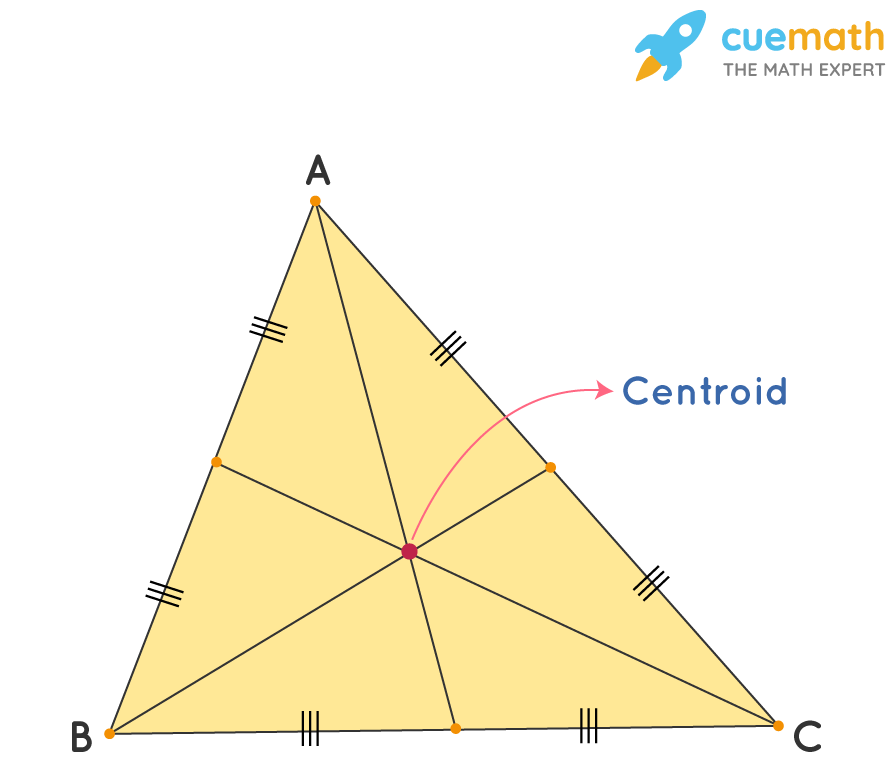# Centroid of A Triangle

## Question: What is the centroid of a triangle?

The geometric center of the object is known as the centroid. It is the point of concurrency of medians of a triangle.

## Answer: The centroid is the center of gravity of the triangle.

The centroid is the point where the medians meet in a the triangle.

## Explanation:

The centroid of a triangle is the center of the triangle, which can be determined as the point of intersection of all the three medians of a triangle.

The centroid divides each median into two parts, which are always in the ratio 2:1.

The centroid formula is used to determine the coordinates of a triangle’s centroid.